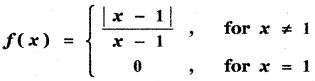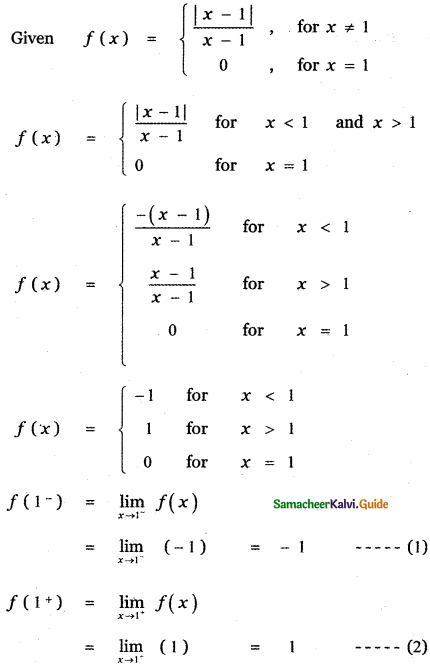Tamilnadu State Board New Syllabus Samacheer Kalvi 11th Maths Guide Pdf Chapter 9 Limits and Continuity Ex 9.1 Text Book Back Questions and Answers, Notes.

## Tamilnadu Samacheer Kalvi 11th Maths Solutions Chapter 9 Limits and Continuity Ex 9.1

In Problems 1 – 6, complete the table using calculator and use the result to estimate the limit.

Question 1.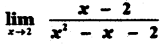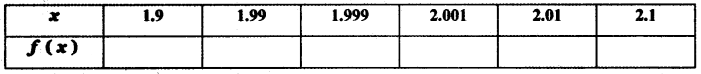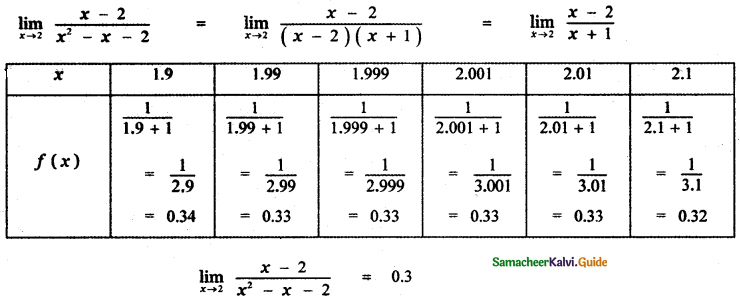Question 2.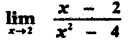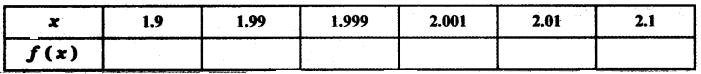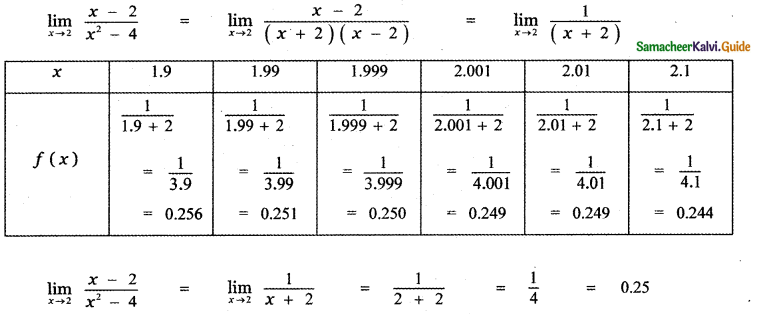Question 3.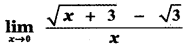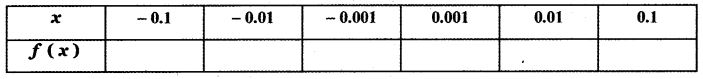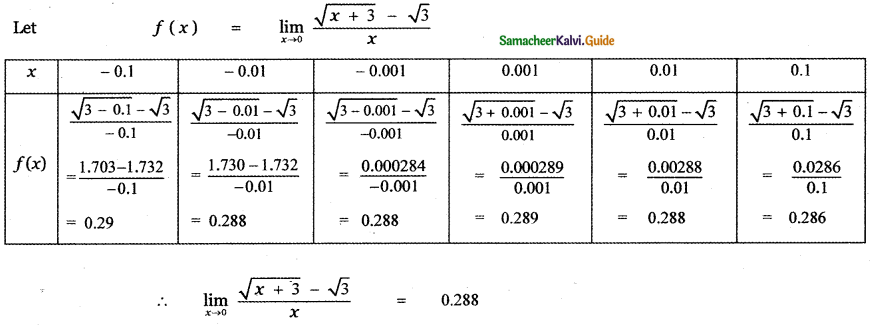Question 4.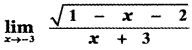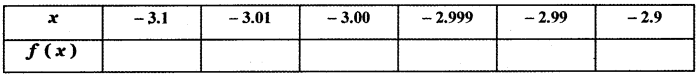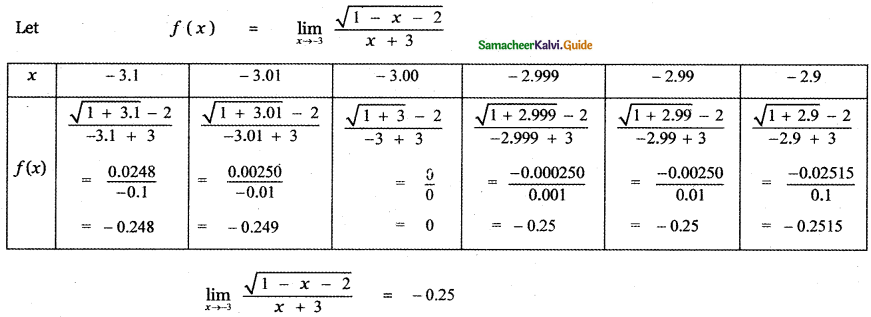Question 5.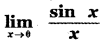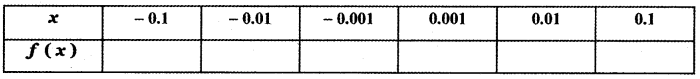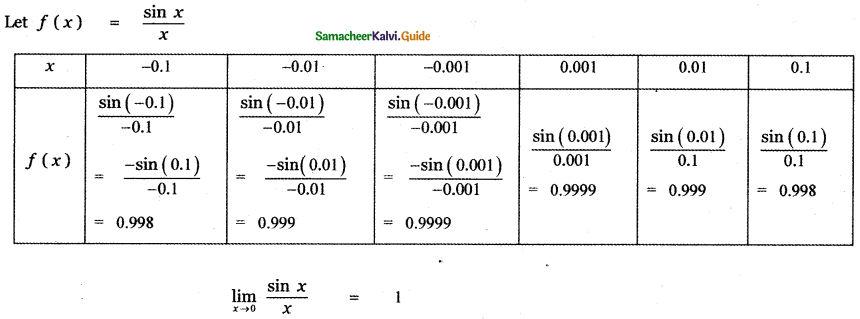Question 6.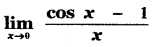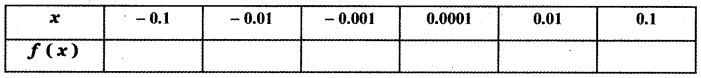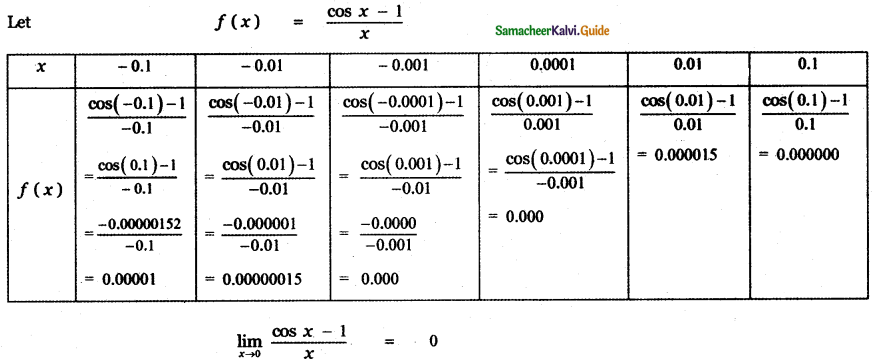Question 7.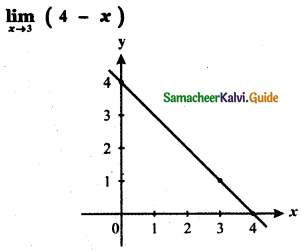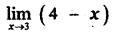From the graph the value of the function at x = 3 is y = f(3) = 1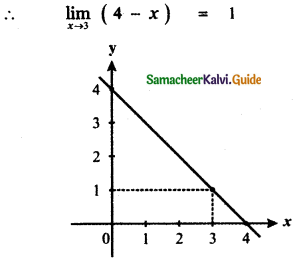Question 8.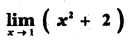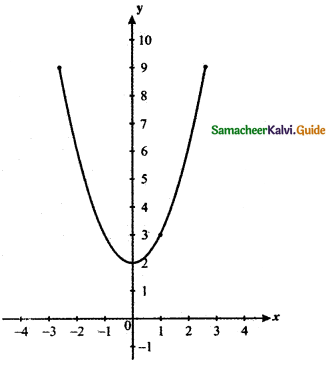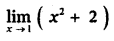From the graph the value of the function at x = 1 is y = f(1) = 3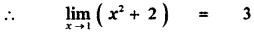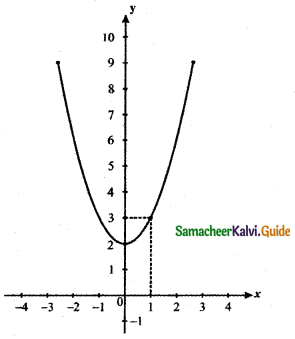Question 9.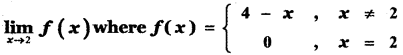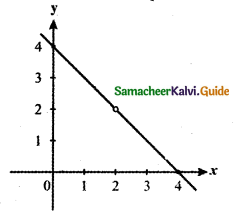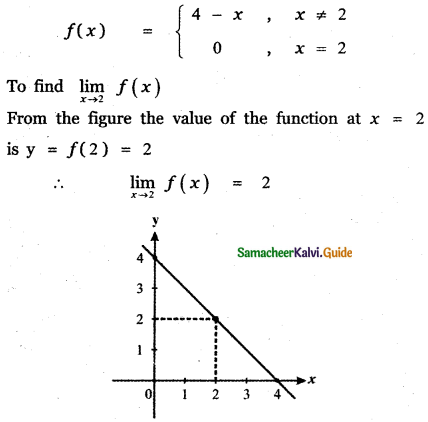Question 10.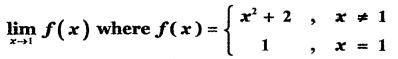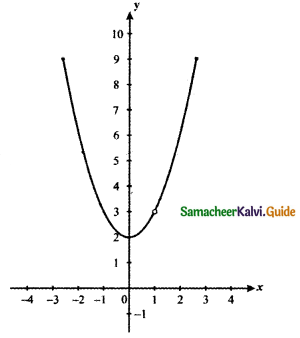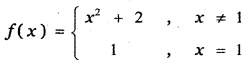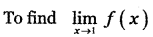From the graph the value of the function is y = f(1) = 3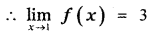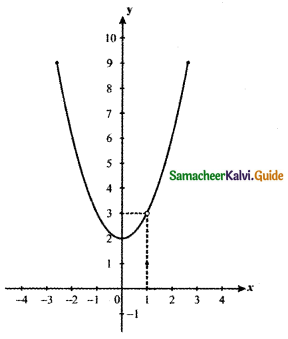Question 11.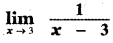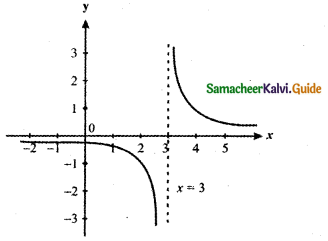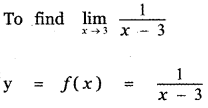From the graph the value of the function at x = 3 the curve does not meet the line x = 3
∴ The value of the function is not defined at the point x = 3.
Hence $$\lim _{x \rightarrow 3} \frac{1}{x-3}$$ does not exist at x = 3.

Question 12.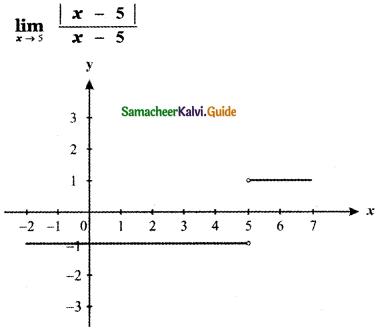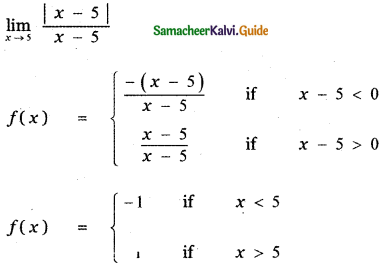From the graph x = 5 curve does not intersect the line x = 5
∴ The value of the function y = f(x) does not exist at x = 5.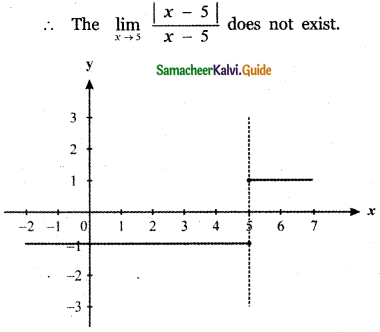Question 13.
$$\lim _{x \rightarrow 1}$$ sin πx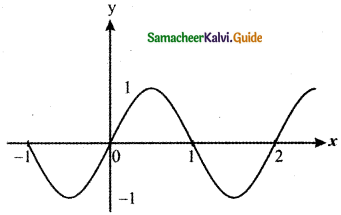$$\lim _{x \rightarrow 1}$$ sin πx
From the graph x = 1, the curve y = f(x) intersects the line x = 1 at x – axis.
∴ y = f(1) = 0
Hence $$\lim _{x \rightarrow 1}$$ sin πx = 0

Question 14.
$$\lim _{x \rightarrow 0}$$ sec x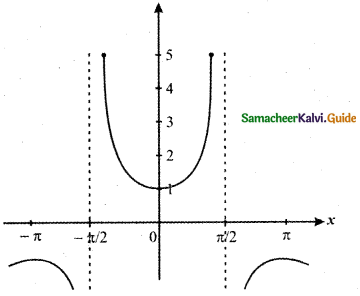To find $$\lim _{x \rightarrow 0}$$ sec x
Let y = f(x) = sec x
From the graph at x = 0 the curve intersect the y – axis.
At x = 0 we have y = 1
∴ $$\lim _{x \rightarrow 0}$$ sec x = 1Question 15.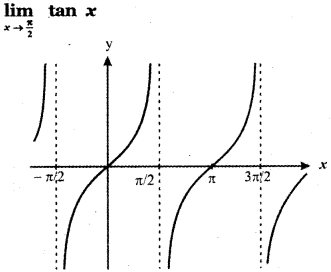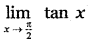y = f(x) = sec x
From the graph at x = $$\frac{\pi}{2}$$, the curve does not intersect the line x = $$\frac{\pi}{2}$$
At x = $$\frac{\pi}{2}$$, the value of the function y = f(x) does not exist.
Hencedoes not exist.

Sketch the graph of f, then identify the values of x0 for which $$\lim _{x \rightarrow x_{0}}$$ f (x) exists.

Question 16.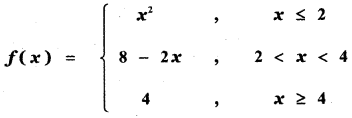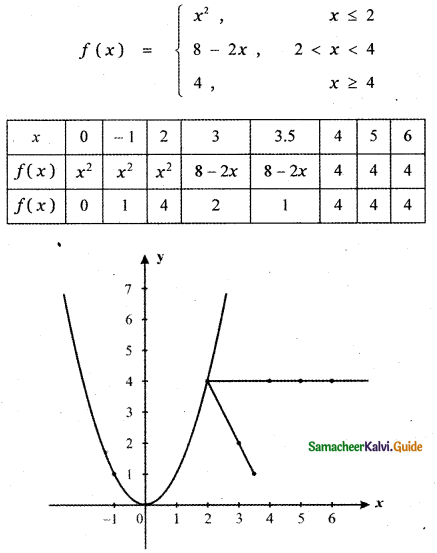At x = 4 , the curve does not exist. Hence, except at x0 = 4 , the limit of f(x) exists.Question 17.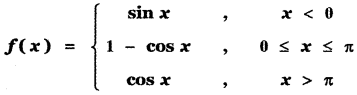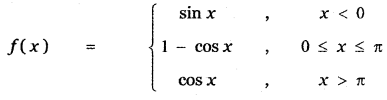From the figure when x = π, y = f(π) = 2. The function is not defined at x = π since sin x lies in the interval [ – 1, 1]
∴ The given function has limits at all points except at x = π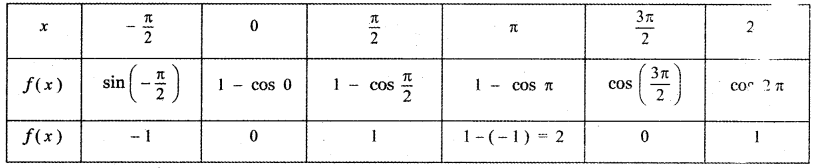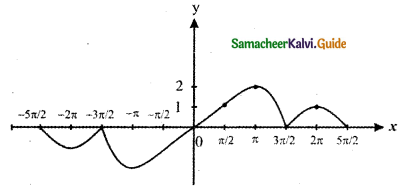(π, 2) point is not possible since the range of the curve is [- 1 , 1] . Except x0 = π, the curve has limits.Question 18.
Sketch the graph of a function f that satisfies the given values:
(i) f(0) is defined
$$\lim _{x \rightarrow 0}$$ f(x) = 4
f(2) = 6
$$\lim _{x \rightarrow 2}$$ f(x) = 3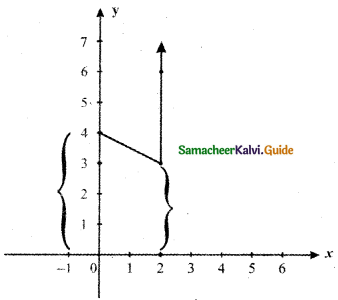(ii) f(-2) = 0
f(2) = 0
$$\lim _{x \rightarrow-2}$$ f(x) = 0
$$\lim _{x \rightarrow 2}$$ f(x) does not exist.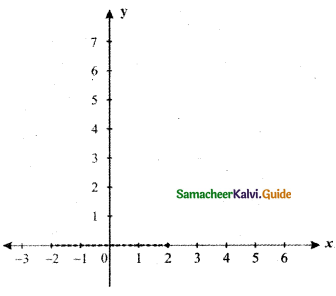Question 19.
Write a brief description of the meaning of the notation $$\lim _{x \rightarrow 8}$$ f(x) = 25
Given $$\lim _{x \rightarrow 8}$$ f(x) = 25
By the definition of limit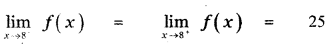∴ f(8) = f(8+) = 25

Question 20.
If f(2) = 4, can you conclude anything about the limit of f (x) as x approaches 2?
No, f(x) = 4, It is the value of the function at x = 2
This limit doesn’t exists at x = 2
Since f(2) = 4
It need not imply that $$\lim _{x \rightarrow 2^{-}} f(x)=\lim _{x \rightarrow 2^{+}} f(x)$$
∴ we cannot conclude at x = 2

Question 21.
If the limit of f (x) as x approaches 2 is 4, can you conclude anything about f (2)? Explain reasoning.
$$\lim _{x \rightarrow 2}$$ f(x) 4 , $$\lim _{x \rightarrow 2^{-}}$$ f(x) = $$\lim _{x \rightarrow 2^{+}}$$ f(x) = 4
When x approaches 2 from the left or from the right f(x) approaches 4.
Given that $$\lim _{x \rightarrow 2^{-}}$$ f(x) = $$\lim _{x \rightarrow 2^{+}}$$ f(x) = 4
The existence or non-existence at x =2 has no leaving on the existence of the limit of f(x) as x approaches to 2.
∴ We cannot conclude the value of f(2).

Question 22.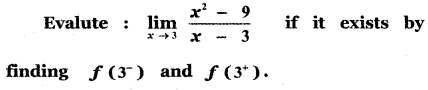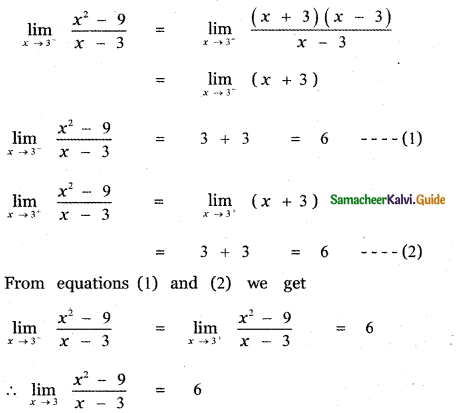Verify the existence of $$\lim _{x \rightarrow 0}$$ f(x), where